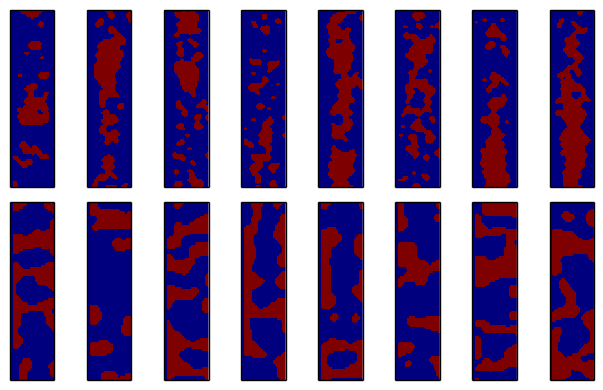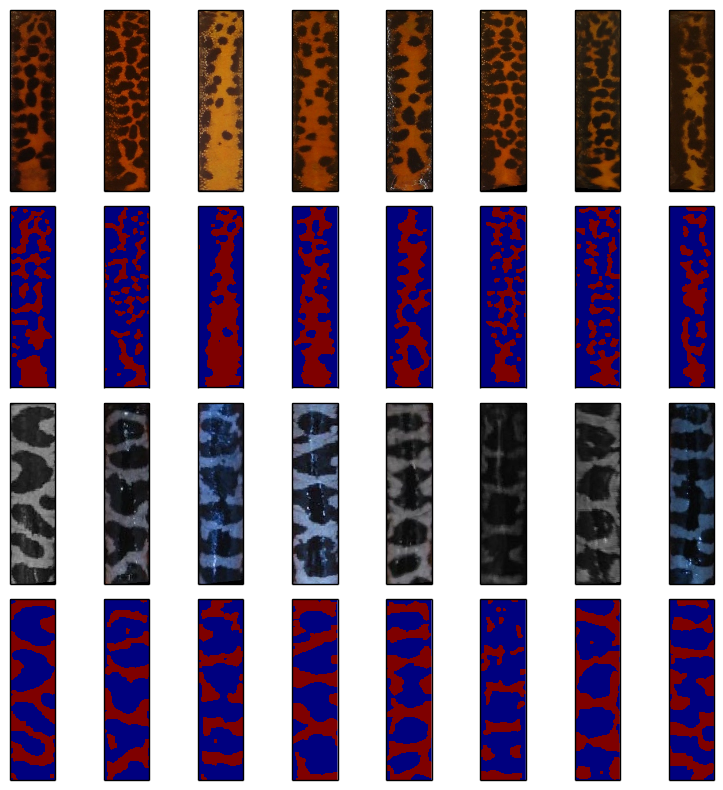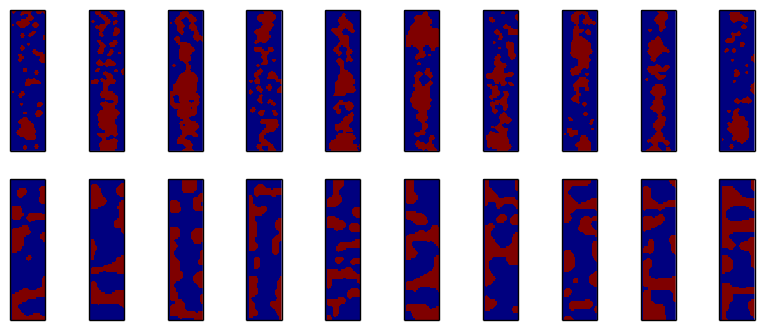# Artificial Spot Pattern Generation by Machine Learning for Photo-identification of Amphibians

Generating artificial spot patterns to analyze the matching performance of algorithms for image recognition of wildlife can be a viable alternative to relying on huge databases of real patterns with known ground truth. In this article we improve the ability of Markov chain based methods for generating artificial spot patterns that resemble the pattern character of a given database.

In the previous article, we have seen that the trained model is not very robust. Since the model only considers the last 60 points in the (linearized) pattern, there is no explicit information about the position in the pattern of the current pixel. As a consequence, it happens that the red spot pattern was not centered or even wrapped around the edges, such that the red area occured at the sides of the pattern. These patterns were not very realistic.

We can improve the definition of the model by inserting markers that determine the beginning of each pixel row. This trick provides explicit knowledge, which column a given pixel belongs to and the generated patterns will be more realistic.

For illustration, let us sample some random patterns from two newly trained models (Crested Newt and Marbled Salamander) using the improved algorithm:

N = 8
figure(figsize=(6.5,4))
for i in range(N):
subplot(2,N,i+1)
Im_full = createPattern(O60_KM)
imshow(Im_full, interpolation='none'); xticks([]); yticks([]);
for i in range(N):
subplot(2,N,i+1+N)
Im_full = createPattern(O60_MSa)
imshow(Im_full, interpolation='none'); xticks([]); yticks([]);
plt.tight_layout()The models are trained from our Crested newt database. Additionally, we train another model with patterns from the Marbled salamander. Some example pictures along with their binarization are shown below:

imgInds = [1, 10, 51, 55, 156, 190, 202, 210]
N = len(imgInds)
files = glob.glob(baseDir_KM + '*.jpg')
el = cv2.getStructuringElement(cv2.MORPH_ELLIPSE, (6,6))
figure(figsize=(8,8))
for i, ind in enumerate(imgInds):
BW = cv2.morphologyEx(ai.thresh_BasedOnMaximumOfAverage(img), cv2.MORPH_OPEN, el)

subplot(4, N, 1+i); imshow(img); xticks([]); yticks([])
subplot(4, N, 1+N+i); imshow(BW, interpolation='none'); xticks([]); yticks([]);

files = glob.glob(baseDir_MSa + '*.jpg')
el = cv2.getStructuringElement(cv2.MORPH_ELLIPSE, (6,6))
for i, ind in enumerate(imgInds):
BW = cv2.morphologyEx(ai.thresh_OtsuOnGrayscale(img), cv2.MORPH_OPEN, el)

subplot(4, N, 2*N+1+i); imshow(img); xticks([]); yticks([])
subplot(4, N, 2*N+1+N+i); imshow(BW, interpolation='none'); xticks([]); yticks([]);
plt.tight_layout()#ignore
import numpy as np
import sys, os
import glob
import cv2
import matplotlib.pyplot as plt

sys.path.append("/home/mmatthe/temp/builds/ai/src/PythonExtensions")
import amphident as ai

%matplotlib inline
from pylab import *

baseDir_KM = '/home/mmatthe/programming/AmphIdent_misc/newts/KMData/'
baseDir_MSa = '/home/mmatthe/programming/AmphIdent_misc/marbled_full/'


We again define the size of the patterns and a downscaled version to reduce the model complexity.

size = 80*320
sizeT = (80, 320)
downScale = 4

size = size / (downScale * downScale)
sizeT = tuple(x/downScale for x in sizeT)


We load all the patterns in the databases and convert them to a binary representation. Note that the first column of each pattern is explicitely set to the value '3', indicating that this is the first column of the pattern. Finall, all patterns are put together into a single string. Each pattern is separated by a several values '2', to determine the beginning and end of each pattern.

def loadDataStr(baseDir):
files = glob.glob(baseDir + '*.jpg')
thresh = ai.thresh_BasedOnMaximumOfAverage if "marbled" not in baseDir else ai.thresh_OtsuOnGrayscale

el = cv2.getStructuringElement(cv2.MORPH_ELLIPSE, (3,3))
count = len(files)
patStart = 4*sizeT
data = np.zeros((count, patStart+size), dtype=np.uint8)
for i, f in enumerate(files):
imgR = cv2.resize(img, sizeT)
BW = thresh(imgR)
BW = cv2.morphologyEx(BW, cv2.MORPH_OPEN, el)
BW /= 255
BW[:,0] = 3 # Indicate the first column of the pattern
data[i,:patStart] = 2  # Indicate a marking between patterns
data[i,patStart:] = BW.flatten()
data_str = "".join(str(x) for x in data.flatten())
return data_str


We again define the function that calculates the transition probabilities of our Markov chain. This function is used to train the model.

from collections import *

def train_char_lm(data, order=4):
lm = defaultdict(Counter)
for i in xrange(len(data)-order):
history, char = data[i:i+order], data[i+order]
lm[history][char]+=1
def normalize(counter):
s = float(sum(counter.values()))
return [(c,cnt/s) for c,cnt in counter.iteritems()]
outlm = {hist:normalize(chars) for hist, chars in lm.iteritems()}
return outlm


We now train the Markov model with the databases of the Crested newt and Marbled salamander. The order of 60 denotes that the last 3 rows of pixels are considered for the suggestion of the following pixel.

O60_KM = train_char_lm(data_str_KM, order=60)
O60_MSa = train_char_lm(data_str_MSa, order=60)


We define the function to calculate the following pixels given a sequence of previous pixels. To keep the model consistent, we deterministically set the pixel to '3', when we are at the left edge of the pattern. In case, the given history does not exist, we simply use a '0' for the next pixel.

from random import random

def generate_letter(lm, history, order):
history = history[-order:]
try:
dist = lm[history]
x = random()
for c,v in dist:
x = x - v
if x <= 0: return c
except KeyError:
return "0"

def generate_text(lm, order, nletters=1000):
history = "2" * order
out = []
for i in xrange(nletters):
if i % sizeT == 0:
c = '3'
else:
c = generate_letter(lm, history, order)
history = history[-order:] + c
out.append(c)
return "".join(out)


Finally, we need to transform the string representation into a binary pattern: We convert all strings to integer and reshape it to the expected pattern size. Additionally, the first column is explicitely set to zero, to remove the artificial '3' from the pattern.

def txtToIm(text):
ints = [int(x) for x in text if x != "2"]
rows = len(ints) / sizeT
ints = ints[:(rows*sizeT)]
BW = np.array(ints).reshape((rows, sizeT))
BW[:,0] = 0
BW = cv2.resize(BW.astype(np.uint8), (80, rows*downScale))
el = cv2.getStructuringElement(cv2.MORPH_ELLIPSE, (5,5))
BW = cv2.morphologyEx(BW, cv2.MORPH_OPEN, el)
return BW

def createPattern(model):
Im = txtToIm(generate_text(model, 60, nletters=2*size))
starty = 0;
Im_full = Im[starty:(starty+320), :]
return Im_full



Now, the model can be used to produce some patterns from Crested newts and Marbled Salamanders:

N = 10
figure(figsize=(10,4))
for i in range(N):
subplot(2,N,i+1)
Im_full = createPattern(O60_KM)
imshow(Im_full, interpolation='none'); xticks([]); yticks([]);
for i in range(N):
subplot(2,N,i+1+N)
Im_full = createPattern(O60_MSa)
imshow(Im_full, interpolation='none'); xticks([]); yticks([]);Obviously, compared to the previous algorithm, the patterns have significantly improved. The crested newt patterns are nicely centered and show areas of smaller spots as well as large areas of constant color. In contrast, when trained with a different pattern database, such as from the marbled salamander, the created patterns are significantly different from the crested newt patterns: They are not necessarily centered and consist of larger regions of equal pixels. Naturally, this is due to the training data, as the marbled salamander has a very different pattern than the crested newt.

Mo, 10 Okt 2016 - Maximilian Matthe